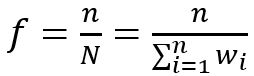United States Department of LaborThe .gov means it's official.
Federal government websites often end in .gov or .mil. Before sharing sensitive information, make sure you're on a federal government site.The site is secure.
The https:// ensures that you are connecting to the official website and that any information you provide is encrypted and transmitted securely.

Injuries, Illnesses, and Fatalities

Contact Us# Survey of Occupational Injuries and Illnesses (SOII) Variance Estimation | November 2021

Variance estimation methodology for the Survey of Occupational Injuries and Illnesses (SOII) was updated with the release of reference year 2020 estimates to accommodate survey weighting that reflects establishment employment size changes and increased nonresponse that are encountered in the current survey environment. To evaluate the impact of the update on the published SOII measure of dispersion, SOII 2019 relative standard errors and publishable injury and illness case counts were compared to estimates created using the updated methodology. While there were minor differences in RSEs and the publishability of some case counts, the update resulted in limited impact on SOII estimates.

The application of the new variance formula does not represent a break in series. The updated estimation methodology is as follows:

The SOII sample design employs a stratified random sampling method to select establishments. The sampling strata are formed by state, ownership, size and targeted estimation industry (TEI). Within a stratum, a simple random sample is drawn. An unbiased variance estimate of a random sample with weights within a SOII stratum is calculated as follows 1:where

• yi is the observed value (e.g., work hours, or count of injuries or illness) of the individual sample unit, i, within a stratum
• wi is the final sample weight of the sample unit, i
• n is the stratum sample size
• ȳ* is the weighted sample meanIn the context of survey sample variance estimation, the finite population correction (FPC) factor is often incorporated into variance estimation, so that the estimated variance is only applied to the portion of the population that is not in the sample. Thus, the variance for the weighted sample mean becomesThe FPC factor is 1-ƒ and the sample fraction 2.

The variance for the weighted sample total (ŷ = Nȳ*) isThe variance of the rate/ratio involving two survey variables (x,y), , following Taylor Series linearization method, is calculated approximately as follows 3:where

• is the total weighted estimate of the variable (case counts for each case type):• is the total weighted estimate of the variable (hours):• var(x̂) is the variance estimate of the variable (total case counts for each case type ())

• var(ŷ) is the variance estimate of the variable (total hours ())

• cov(x̂,ŷ) is the covariance estimate between two variables (case counts of each case type and hours)## Footnotes:

2. Cochran, W. G. (1977). Sampling techniques (3rd ed.). Wiley. p.24

3. Lohr, S. L. (2010). Sampling: Design and Analysis, Second edition. Brooks/Cole, Cengage Learning.